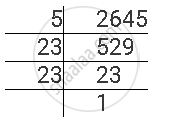Share

# For the Following Number, Find the Smallest Whole Number by Which It Should Be Divided So as to Get a Perfect Square Number. Also Find the Square Root of the Square Number So Obtained. 2645 - CBSE Class 8 - Mathematics

ConceptSquare Roots - Finding Square Root Through Prime Factorisation

#### Question

For the following number, find the smallest whole number by which it should be divided so as to get a perfect square number. Also find the square root of the square number so obtained.

2645

#### Solution

2645 can be factorised as follows.2645 =  5 x 23 x 23

Here, prime factor 5 does not have its pair.

If we divide this number by 5, then the number will become a perfect square.

Therefore, 2645 has to be divided by 5 to obtain a perfect square.

2645 ÷5 = 529 is a perfect square.

529 = 23 x 23

∴ sqrt529 = 23

Is there an error in this question or solution?

#### APPEARS IN

NCERT Solution for Mathematics Textbook for Class 8 (2018 to Current)
Chapter 6: Squares and Square Roots
Ex. 6.3 | Q: 6.4 | Page no. 102
Solution For the Following Number, Find the Smallest Whole Number by Which It Should Be Divided So as to Get a Perfect Square Number. Also Find the Square Root of the Square Number So Obtained. 2645 Concept: Square Roots - Finding Square Root Through Prime Factorisation.
S